Next: Example 5.4: Electric potential due Up: Electric Potential Previous: Example 5.2: Motion of an

## Example 5.3: Electric potential due to point charges

Question:  A particle of charge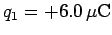is located on the-axis at the point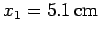. A second particle of charge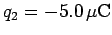is placed on the-axis at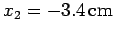. What is the absolute electric potential at the origin (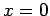)? How much work must we perform in order to slowly move a charge of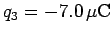from infinity to the origin, whilst keeping the other two charges fixed?

Solution: The absolute electric potential at the origin due to the first charge is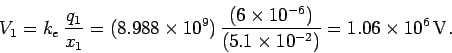Likewise, the absolute electric potential at the origin due to the second charge is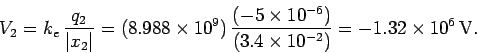The net potentialat the origin is simply the algebraic sum of the potentials due to each charge taken in isolation. Thus,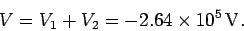The work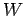which we must perform in order to slowly moving a charge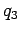from infinity to the origin is simply the product of the charge and the potential differencebetween the end and beginning points. Thus,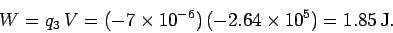Next: Example 5.4: Electric potential due Up: Electric Potential Previous: Example 5.2: Motion of an
Richard Fitzpatrick 2007-07-14